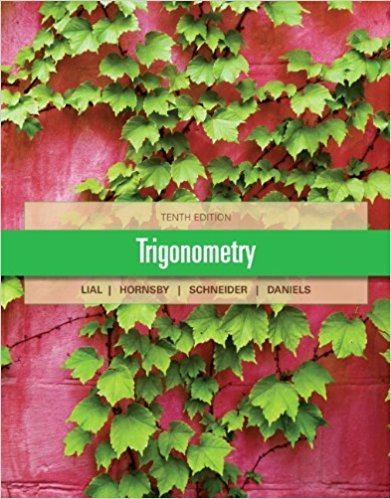×
×

# Solutions for Chapter 8.1: Complex Numbers## Full solutions for Trigonometry | 10th Edition

ISBN: 9780321671776Solutions for Chapter 8.1: Complex Numbers

Solutions for Chapter 8.1
4 5 0 320 Reviews
30
0
##### ISBN: 9780321671776

Chapter 8.1: Complex Numbers includes 116 full step-by-step solutions. This expansive textbook survival guide covers the following chapters and their solutions. Since 116 problems in chapter 8.1: Complex Numbers have been answered, more than 35672 students have viewed full step-by-step solutions from this chapter. Trigonometry was written by and is associated to the ISBN: 9780321671776. This textbook survival guide was created for the textbook: Trigonometry, edition: 10.

Key Math Terms and definitions covered in this textbook
• Augmented matrix [A b].

Ax = b is solvable when b is in the column space of A; then [A b] has the same rank as A. Elimination on [A b] keeps equations correct.

• Cofactor Cij.

Remove row i and column j; multiply the determinant by (-I)i + j •

• Column picture of Ax = b.

The vector b becomes a combination of the columns of A. The system is solvable only when b is in the column space C (A).

A sequence of steps (end of Chapter 9) to solve positive definite Ax = b by minimizing !x T Ax - x Tb over growing Krylov subspaces.

• Echelon matrix U.

The first nonzero entry (the pivot) in each row comes in a later column than the pivot in the previous row. All zero rows come last.

• Elimination.

A sequence of row operations that reduces A to an upper triangular U or to the reduced form R = rref(A). Then A = LU with multipliers eO in L, or P A = L U with row exchanges in P, or E A = R with an invertible E.

• Fourier matrix F.

Entries Fjk = e21Cijk/n give orthogonal columns FT F = nI. Then y = Fe is the (inverse) Discrete Fourier Transform Y j = L cke21Cijk/n.

• Hessenberg matrix H.

Triangular matrix with one extra nonzero adjacent diagonal.

• Kronecker product (tensor product) A ® B.

Blocks aij B, eigenvalues Ap(A)Aq(B).

• lA-II = l/lAI and IATI = IAI.

The big formula for det(A) has a sum of n! terms, the cofactor formula uses determinants of size n - 1, volume of box = I det( A) I.

• Length II x II.

Square root of x T x (Pythagoras in n dimensions).

• Linear transformation T.

Each vector V in the input space transforms to T (v) in the output space, and linearity requires T(cv + dw) = c T(v) + d T(w). Examples: Matrix multiplication A v, differentiation and integration in function space.

• Normal equation AT Ax = ATb.

Gives the least squares solution to Ax = b if A has full rank n (independent columns). The equation says that (columns of A)·(b - Ax) = o.

• Nullspace N (A)

= All solutions to Ax = O. Dimension n - r = (# columns) - rank.

• Row space C (AT) = all combinations of rows of A.

Column vectors by convention.

• Skew-symmetric matrix K.

The transpose is -K, since Kij = -Kji. Eigenvalues are pure imaginary, eigenvectors are orthogonal, eKt is an orthogonal matrix.

• Subspace S of V.

Any vector space inside V, including V and Z = {zero vector only}.

• Vandermonde matrix V.

V c = b gives coefficients of p(x) = Co + ... + Cn_IXn- 1 with P(Xi) = bi. Vij = (Xi)j-I and det V = product of (Xk - Xi) for k > i.

v + w = (VI + WI, ... , Vn + Wn ) = diagonal of parallelogram.

• Volume of box.

The rows (or the columns) of A generate a box with volume I det(A) I.

×